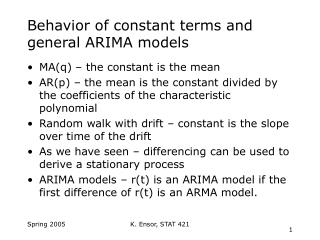# Behavior of constant terms and general ARIMA models - PowerPoint PPT PresentationDownload PresentationBehavior of constant terms and general ARIMA models

Behavior of constant terms and general ARIMA models
Download Presentation## Behavior of constant terms and general ARIMA models

- - - - - - - - - - - - - - - - - - - - - - - - - - - E N D - - - - - - - - - - - - - - - - - - - - - - - - - - -
##### Presentation Transcript

1. Behavior of constant terms and general ARIMA models • MA(q) – the constant is the mean • AR(p) – the mean is the constant divided by the coefficients of the characteristic polynomial • Random walk with drift – constant is the slope over time of the drift • As we have seen – differencing can be used to derive a stationary process • ARIMA models – r(t) is an ARIMA model if the first difference of r(t) is an ARMA model. K. Ensor, STAT 421

2. Unit-root nonstationary • Random walk p(t)=p(t-1)+a(t) p(0)=initial value a(t)~WN(0,2) • Often used as model for stock movement (logged stock prices). • Nonstationary • The impact of past shocks never diminishes – “shocks are said to have a permanent effect on the series”. • Prediction? • Not mean reverting • Variance of forecast error goes to infinity as the prediction horizon goes to infinity K. Ensor, STAT 421

3. K. Ensor, STAT 421

4. K. Ensor, STAT 421

5. Random Walk with Drift • Include a constant mean in the random walk model. • Time-trend of the log price p(t) and is referred to as the drift of the model. • The drift is multiplicative over time p(t)=t + p(0) + a(t) + … + a(1) • What happens to the variance? K. Ensor, STAT 421

6. Drift parameter= 0.5 Standard Deviation of shocks=2.0 K. Ensor, STAT 421

7. Drift parameter= 0.5 Standard Deviation of shocks=2.0 K. Ensor, STAT 421

8. Unit Root Tests • The classic test was derived by Dickey and Fuller in 1979. The objective is to test the presence of a unit root vs. the alternative of a stationary model. • The behavior of the test statistics differs if the null is a random walk with drift or if it is a random walk without drift (see text for details). K. Ensor, STAT 421

9. Unit root tests continued • There are many forms. The easiest to conceptualize is the following version of the Augmented Dickey Fuller test (ADF): • The test for unit roots then is simply a test of the following hypothesis: against • Use the usual t-statistic for testing the null hypothesis. Distribution properties are different. K. Ensor, STAT 421

10. Unit root tests • In finmetrics use the following command • Without finmetrics you will need to simulate the distribution under the null hypothesis – see the Zivot manual for the algorithm. • unitroot(rseries,trend="c",statistic="t", method="adf",lags=6) K. Ensor, STAT 421

11. Stationary Tests • Null hypothesis is that of stationarity. • Alternative is a non-stationary process. • Null hypothesis is that the variance of ε is 0. • In finmetrics use command stationaryTest(x, trend="c", bandwidth=NULL, na.rm=F) K. Ensor, STAT 421